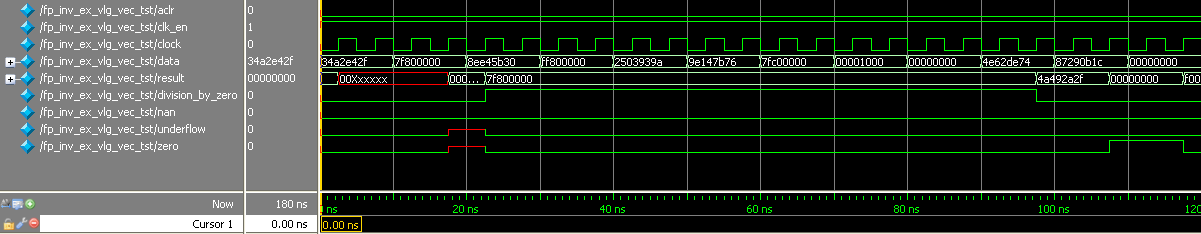ID 683750
Date 9/13/2021
Public

## 8.5.1. ALTFP_INV Design Example: Understanding the Simulation Results

The simulation waveform in this design example is not shown in its entirety. Run the design example files in the ModelSim* - Intel® FPGA Edition software to see the complete simulation waveforms.

These figures show the expected simulation results in the ModelSim* - Intel® FPGA Edition software.

Figure 26. ALTFP_INV Simulation Waveform (Input Data)Figure 27. ALTFP_INV Simulation Waveform (Output Data)This design example implements a floating-point inverse for single-precision format numbers. The optional input ports (clk_en and aclr) and all four exception handling output ports (division_by_zero, nan, zero, and underflow) are enabled.

The latency is fixed at 20 clock cycles; therefore, every inverse operation outputs results 20 clock cycles later.

This table lists the inputs and corresponding outputs obtained from the simulation in the waveforms.

Table 47.  Summary of Input Values and Corresponding Outputs
Time Event
0 ns, start-up data[] value: 34A2 E42Fh

Output value: An undefined value is seen on the result[] port, which is ignored. All values seen on the output port before the 20th clock cycle are merely due to the behavior of the system during start-up and should be disregarded.

97.5 ns Output value: 4A49 2A2Fh

Exception handling ports: division_by_zero deasserts

The inverse of a normal number results in a normal value.

10 ns data[] value: 7F80 0000h

This is an infinity value.

107.5 ns Output value: 0000 0000h

Exception handling ports: zero asserts

The inverse of an infinity value produces a zero.

60 ns data[] value: 7FC0 0000h

This is a NaN.

157.5 ns Output value: 7FC0 0000h

Exception handling ports: nan asserts

The inverse of a NaN results in a NaN

70 ns data[] value: 0000 1000h

This is a denormal number.

167.5 ns Output value: 7F80 0000h

Exception handling ports: nan deasserts, division_by_zero asserts

Denormal numbers are forced-zero values, therefore, the inverse of a zero results in infinity.